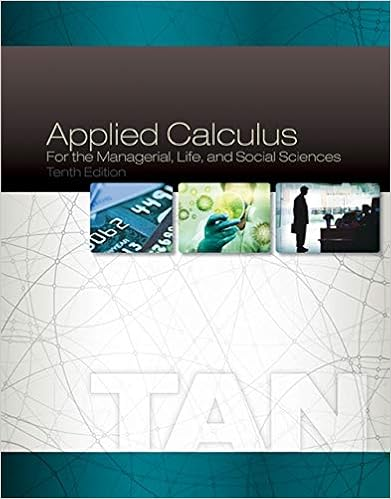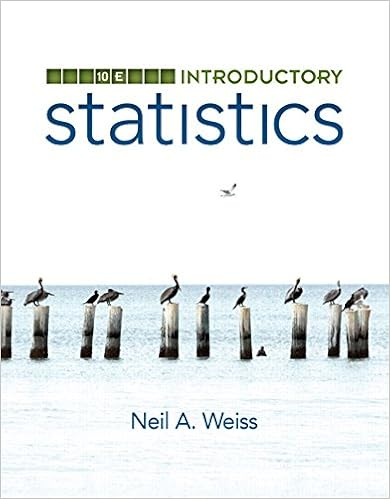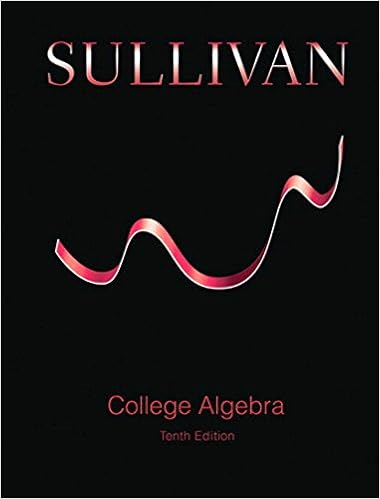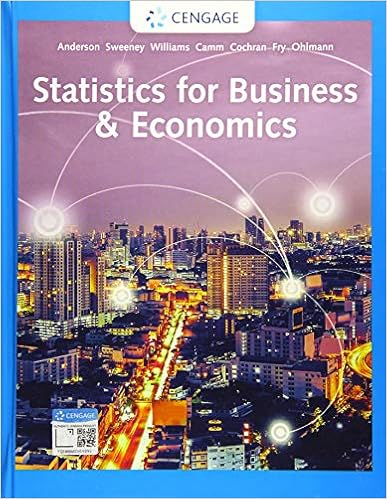# final_2006 - MS&E 221 Ramesh Johari Final Examination March...

• Notes
• DeaconIceJackal9588
• 12

This preview shows page 1 - 3 out of 12 pages.

The preview shows page 1 - 3 out of 12 pages.
MS&E 221Final ExaminationRamesh JohariMarch 22, 2006Instructions1. Take alternate seating if possible.2. Answer all questions in the spaces provided on these sheets. If needed, additional paper will be avail-able at the front of the room. Answers given on any other paper will not be counted.3. You may use no notes, books, or calculators.4.Explain your answers!It helps earn you partial credit.5. The examination begins at 8:30 am, and ends at 11:30 am.Honor CodeIn taking this examination, I acknowledge and accept the Stanford University Honor Code.NAME(signed)NAME(printed)1
##### We have textbook solutions for you!The document you are viewing contains questions related to this textbook.
Chapter 10 / Exercise 36
Applied Calculus for the Managerial, Life, and Social Sciences
TanExpert VerifiedBrowse all Textbook Solutions
Useful Formulas1. IfTis an exponentially distributed random variable with mean1, then the density ofTis given byfT, where:fT(t) =λe-λt,t0.2. IfSis a random variable with a gamma distribution of parametersnandλ, wherenis a positiveinteger, then the density ofSis given byfS, where:fS(s) =λe-λs(λs)n-1(n-1)!,s0.3. For anM/M/1queue with arrival rateλand service rateμwithλ < μ, the equilibrium distributionis:P(Q=j) = (1-ρ)ρj,j= 0,1,2, . . . ,whereρ=λ/μ.4. For anM/M/queue with arrival rateλand service rateμ, the equilibrium distribution is:P(Q=j) =e-ρρjj!,j= 0,1,2, . . . ,whereρ=λ/μ.5. For anM/M/K/Kqueue with arrival rateλand service rateμ, the equilibrium distribution is:P(Q=j) =ρj/j!1 +· · ·+ρK/K!,j= 0,1,2, . . . , K,whereρ=λ/μ.2

Course Hero member to access this document

Course Hero member to access this document

End of preview. Want to read all 12 pages?

Course Hero member to access this document

Term
Winter
Professor
Ramesh
Tags
Probability theory, Markov chain, time Markov chain
##### We have textbook solutions for you!
The document you are viewing contains questions related to this textbook.The document you are viewing contains questions related to this textbook.
Chapter 10 / Exercise 36
Applied Calculus for the Managerial, Life, and Social Sciences
TanExpert Verified
•••